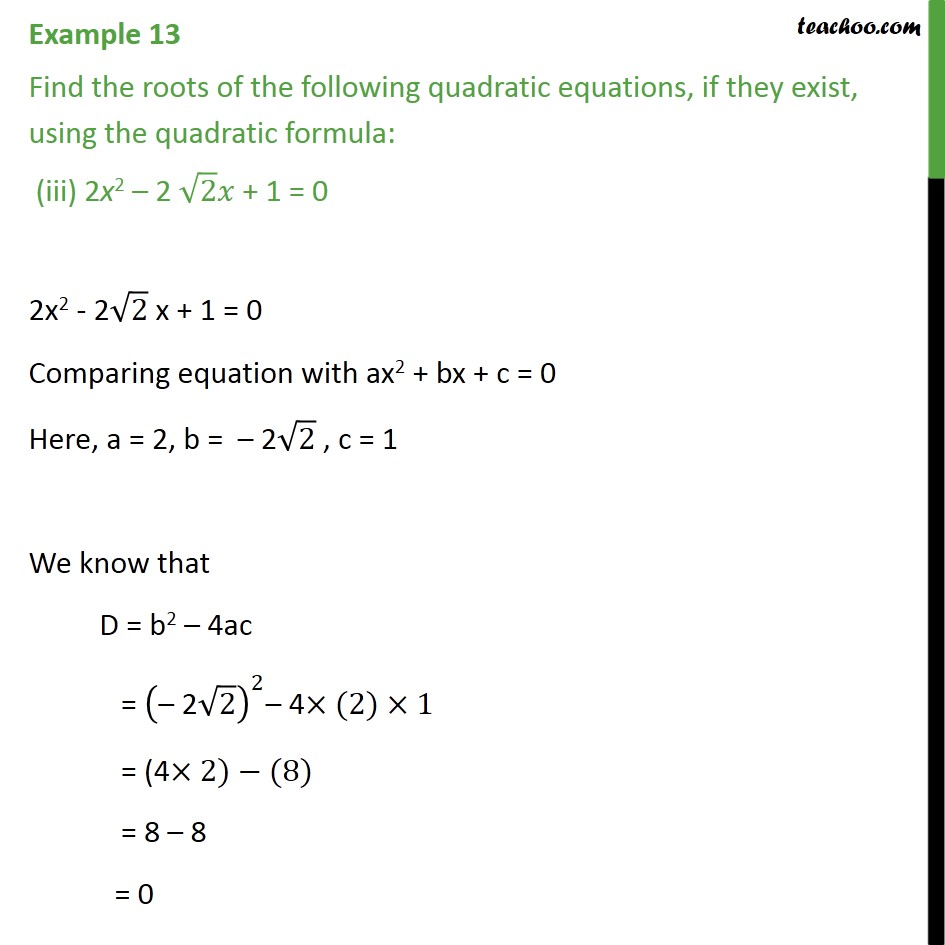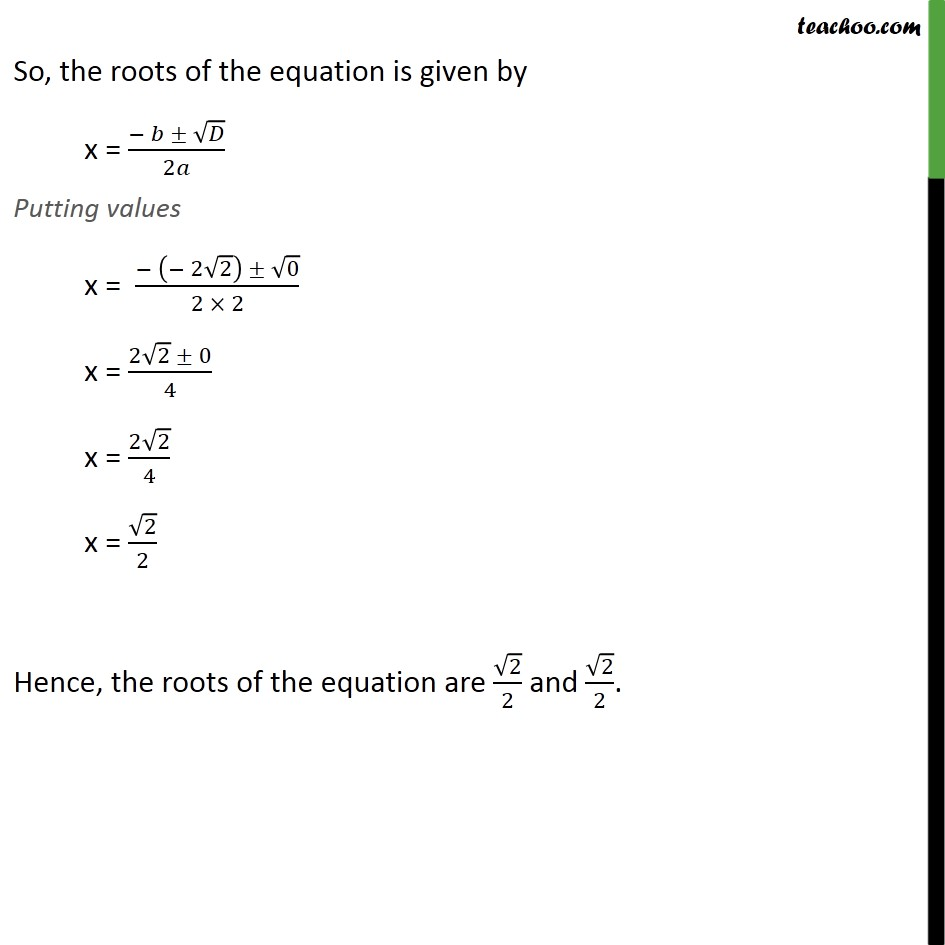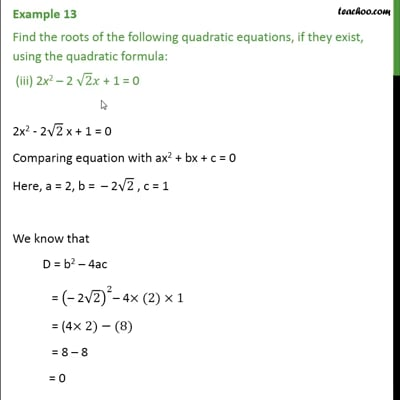Examples

Chapter 4 Class 10 Quadratic Equations (Term 2)
Serial order wiseThis video is only available for Teachoo black users

### Transcript

Example 13 Find the roots of the following quadratic equations, if they exist, using the quadratic formula: (iii) 2x2 2 2 + 1 = 0 2x2 - 2 2 x + 1 = 0 Comparing equation with ax2 + bx + c = 0 Here, a = 2, b = 2 2 , c = 1 We know that D = b2 4ac = (" 2" 2)^2 4 (2) 1 = (4 2) (8) = 8 8 = 0 So, the roots of the equation is given by x = ( )/2 Putting values x = ( ( 2 2) 0)/(2 2) x = (2 2 0)/4 x = (2 2)/4 x = 2/2 Hence, the roots of the equation are 2/2 and 2/2.